# Quadruple Boson Energy Conservation

##### Sebastian Micluța-Câmpeanu, Chris Rackauckas

In this notebook we will study the energy conservation properties of several high-order methods for a system with the following Hamiltonian:

$\mathcal{H}\left(q_0,q_2,p_0,p_2\right) = \frac{A}{2} \left(p_0^2 + p_2^2 + q_0^2 + q_2^2\right) + \frac{B}{\sqrt{2}} q_0 \left(3q_2^2 - q_0^2\right) + \frac{D}{4} \left(q_0^2+q_2^2\right)^2$

This Hamiltonian resembles the Hénon-Heiles one, but it has an additional fourth order term. The aim of this benchmark is to see what happens with the energy error when highly accurate solutions are needed and how the results compare with the Hénon-Heiles case.

using OrdinaryDiffEq, Plots, DiffEqCallbacks, LinearAlgebra
using TaylorIntegration

T(p) = A / 2 * norm(p)^2
V(q) = A / 2 * (q^2 + q^2) + B / √2 * q * (3 * q^2 - q^2) + D / 4 * (q^2 + q^2)^2
H(p, q, params) = T(p) + V(q)

const A, B, D = 1., 0.55, 0.4

module InPlace
using ParameterizedFunctions

function q̇(dq, p, q, params, t)
dq = A * p
dq = A * p
end

function ṗ(dp, p, q, params, t)
dp = -A * q - 3 * B / √2 * (q^2 - q^2) - D * q * (q^2 + q^2)
dp = -q * (A + 3 * √2 * B * q + D * (q^2 + q^2))
end

const A, B, D = 1., 0.55, 0.4

const q0 = [4.919080920016389, 2.836942666663649]
const p0 = [0., 0.]
const u0 = vcat(p0,q0)

h_eqs = @ode_def HamiltEqs begin
dp₀ = -A * q₀ - 3 * B / √2 * (q₂^2 - q₀^2) - D * q₀ * (q₀^2 + q₂^2)
dp₂ = -q₂ * (A + 3 * √2 * B * q₀ + D * (q₀^2 + q₂^2))
dq₀ = A * p₀
dq₂ = A * p₂
end A B D

p = [1.0,0.55,0.4]

end

module OutOfPlace
using StaticArrays

function q̇(p, q, params, t)
p
end

function ṗ(p, q, params, t)
dp1 = -A * q - 3 * B / √2 * (q^2 - q^2) - D * q * (q^2 + q^2)
dp2 = -q * (A + 3 * √2 * B * q + D * (q^2 + q^2))
@SVector [dp1, dp2]
end

const A, B, D = 1., 0.55, 0.4

const q0 = @SVector [4.919080920016389, 2.836942666663649]
const p0 = @SVector [0., 0.]
const u0 = vcat(p0,q0)

h_eqs(z, params, t) = SVector(
-A * z - 3 * B / √2 * (z^2 - z^2) - D * z * (z^2 + z^2),
-z * (A + 3 * √2 * B * z + D * (z^2 + z^2)),
z,
z
)

p = nothing

end

function g(resid,u)
resid = H([u,u],[u,u],nothing) - E
resid[2:4] .= 0
end

const E = H(InPlace.p0, InPlace.q0, InPlace.p)
const cb = ManifoldProjection(g, nlopts=Dict(:ftol=>1e-13))

DiffEqBase.DiscreteCallback{getfield(DiffEqCallbacks, Symbol("##5#6")),Diff
EqCallbacks.ManifoldProjection{true,DiffEqCallbacks.NonAutonomousFunction{t
ypeof(Main.WeaveSandBox0.g),true},OrdinaryDiffEq.NLSOLVEJL_SETUP{0,true},Di
ct{Symbol,Float64}},typeof(DiffEqCallbacks.Manifold_initialize)}(getfield(D
iffEqCallbacks, Symbol("##5#6"))(), DiffEqCallbacks.ManifoldProjection{true
,DiffEqCallbacks.NonAutonomousFunction{typeof(Main.WeaveSandBox0.g),true},O
rdinaryDiffEq.NLSOLVEJL_SETUP{0,true},Dict{Symbol,Float64}}(DiffEqCallbacks
.NonAutonomousFunction{typeof(Main.WeaveSandBox0.g),true}(Main.WeaveSandBox
0.g, 0, 0), DiffEqCallbacks.NonAutonomousFunction{typeof(Main.WeaveSandBox0
.g),true}(Main.WeaveSandBox0.g, 0, 0), OrdinaryDiffEq.NLSOLVEJL_SETUP{0,tru
e}(), Dict(:ftol=>1.0e-13)), DiffEqCallbacks.Manifold_initialize, Bool[fals
e, true])


For the comparison we will use the following function

energy_err(sol) = map(i->H([sol[1,i], sol[2,i]], [sol[3,i], sol[4,i]],nothing)-E, 1:length(sol.u))
abs_energy_err(sol) = [abs.(H([sol[1,j], sol[2,j]], [sol[3,j], sol[4,j]],nothing) - E) for j=1:length(sol.u)]

function compare(mode=InPlace, all=true, plt=nothing; tmax=1e2)
prob1 = DynamicalODEProblem(mode.ṗ,  mode.q̇, mode.p0, mode.q0, (0., tmax))
prob2 = ODEProblem(mode.h_eqs, mode.u0, (0., tmax), mode.p)

GC.gc()
(mode == InPlace  && all) && @time sol1 = solve(prob2, Vern9(), callback=cb, abstol=1e-14, reltol=1e-14)
GC.gc()
@time sol2 = solve(prob1, KahanLi8(), dt=1e-2, maxiters=1e10)
GC.gc()
@time sol3 = solve(prob1, SofSpa10(), dt=1e-2, maxiters=1e8)
GC.gc()
@time sol4 = solve(prob2, Vern9(), abstol=1e-14, reltol=1e-14)
GC.gc()
@time sol5 = solve(prob1, DPRKN12(), abstol=1e-14, reltol=1e-14)
GC.gc()
(mode == InPlace && all) && @time sol6 = solve(prob2, TaylorMethod(50), abstol=1e-20)

(mode == InPlace && all) && println("Vern9 + ManifoldProjection max energy error:\t"*
"$(maximum(abs_energy_err(sol1)))\tin\t$(length(sol1.u))\tsteps.")
println("KahanLi8 max energy error:\t\t\t$(maximum(abs_energy_err(sol2)))\tin\t$(length(sol2.u))\tsteps.")
println("SofSpa10 max energy error:\t\t\t$(maximum(abs_energy_err(sol3)))\tin\t$(length(sol3.u))\tsteps.")
println("Vern9 max energy error:\t\t\t\t$(maximum(abs_energy_err(sol4)))\tin\t$(length(sol4.u))\tsteps.")
println("DPRKN12 max energy error:\t\t\t$(maximum(abs_energy_err(sol5)))\tin\t$(length(sol5.u))\tsteps.")
(mode == InPlace && all) && println("TaylorMethod max energy error:\t\t\t$(maximum(abs_energy_err(sol6)))"* "\tin\t$(length(sol6.u))\tsteps.")

if plt == nothing
plt = plot(xlabel="t", ylabel="Energy error")
end

(mode == InPlace && all) && plot!(sol1.t, energy_err(sol1), label="Vern9 + ManifoldProjection")
plot!(sol2.t, energy_err(sol2), label="KahanLi8", ls=mode==InPlace ? :solid : :dash)
plot!(sol3.t, energy_err(sol3), label="SofSpa10", ls=mode==InPlace ? :solid : :dash)
plot!(sol4.t, energy_err(sol4), label="Vern9", ls=mode==InPlace ? :solid : :dash)
plot!(sol5.t, energy_err(sol5), label="DPRKN12", ls=mode==InPlace ? :solid : :dash)
(mode == InPlace && all) && plot!(sol6.t, energy_err(sol6), label="TaylorMethod")

return plt
end

compare (generic function with 4 methods)


The mode argument choses between the in place approach and the out of place one. The all parameter is used to compare only the integrators that support both the in place and the out of place versions (we reffer here only to the 6 high order methods chosen bellow). The plt argument can be used to overlay the results over a previous plot and the tmax keyword determines the simulation time.

Note:

1. The Vern9 method is used with ODEProblem because of performance issues with ArrayPartition indexing which manifest for DynamicalODEProblem.

2. The NLsolve call used by ManifoldProjection was modified to use ftol=1e-13 in order to obtain a very low energy error.

Here are the results of the comparisons between the in place methods:

compare(tmax=1e2)

19.625903 seconds (53.01 M allocations: 2.450 GiB, 10.33% gc time)
4.199081 seconds (11.62 M allocations: 552.223 MiB, 7.40% gc time)
2.594057 seconds (6.27 M allocations: 280.569 MiB, 5.64% gc time)
2.558870 seconds (4.54 M allocations: 196.265 MiB, 4.27% gc time)
2.522871 seconds (5.83 M allocations: 290.359 MiB, 6.58% gc time)
1.696476 seconds (4.66 M allocations: 417.098 MiB, 7.69% gc time)
Vern9 + ManifoldProjection max energy error:	1.8474111129762605e-13	in	9727
steps.
KahanLi8 max energy error:			5.5706550483591855e-12	in	10001	steps.
SofSpa10 max energy error:			3.836930773104541e-12	in	10001	steps.
Vern9 max energy error:				1.6058265828178264e-12	in	4864	steps.
DPRKN12 max energy error:			7.105427357601002e-13	in	1907	steps.
TaylorMethod max energy error:			4.831690603168681e-13	in	509	steps.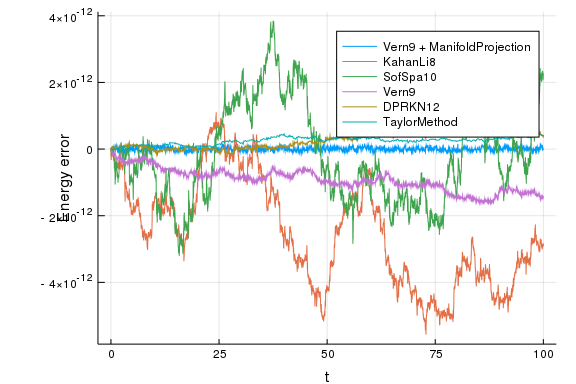compare(tmax=1e3)

0.913728 seconds (3.30 M allocations: 285.846 MiB, 38.62% gc time)
0.240153 seconds (1.50 M allocations: 87.745 MiB, 59.78% gc time)
0.347536 seconds (1.50 M allocations: 87.746 MiB, 56.42% gc time)
0.149748 seconds (632.22 k allocations: 67.646 MiB, 48.24% gc time)
0.043951 seconds (467.44 k allocations: 20.215 MiB)
2.259182 seconds (15.32 M allocations: 2.237 GiB, 30.61% gc time)
Vern9 + ManifoldProjection max energy error:	1.9895196601282805e-13	in	9724
5	steps.
KahanLi8 max energy error:			1.0530243343964685e-11	in	100002	steps.
SofSpa10 max energy error:			1.5077716852829326e-11	in	100002	steps.
Vern9 max energy error:				1.0615508472255897e-11	in	48624	steps.
DPRKN12 max energy error:			4.874323167314287e-12	in	18930	steps.
TaylorMethod max energy error:			1.0089706847793423e-12	in	5082	steps.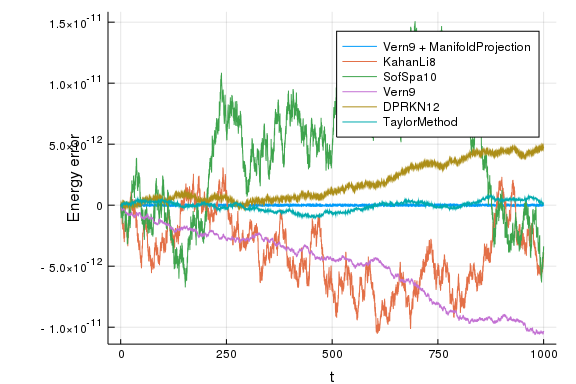compare(tmax=1e4)

6.683562 seconds (32.96 M allocations: 2.780 GiB, 20.01% gc time)
4.410263 seconds (15.00 M allocations: 831.610 MiB, 76.06% gc time)
6.735835 seconds (15.00 M allocations: 831.611 MiB, 77.18% gc time)
7.694149 seconds (6.32 M allocations: 676.280 MiB, 89.68% gc time)
5.648650 seconds (4.67 M allocations: 199.615 MiB, 92.23% gc time)
27.603365 seconds (153.20 M allocations: 22.037 GiB, 39.44% gc time)
Vern9 + ManifoldProjection max energy error:	2.2737367544323206e-13	in	9723
67	steps.
KahanLi8 max energy error:			4.3968384488835e-11	in	1000001	steps.
SofSpa10 max energy error:			6.492939519375795e-11	in	1000001	steps.
Vern9 max energy error:				7.220535280794138e-11	in	486181	steps.
DPRKN12 max energy error:			2.701483481359901e-11	in	189210	steps.
TaylorMethod max energy error:			5.073275133327115e-12	in	50814	steps.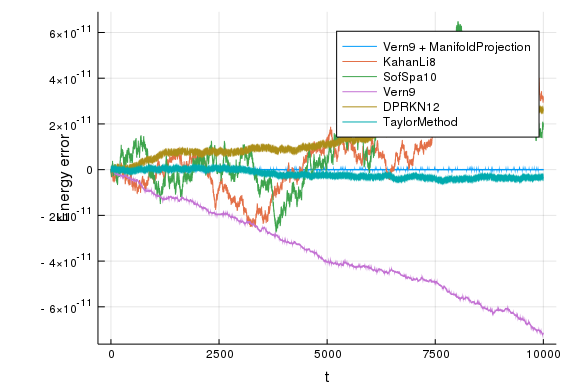compare(tmax=2e4)

15.416573 seconds (65.90 M allocations: 5.551 GiB, 22.52% gc time)
9.998758 seconds (30.00 M allocations: 1.669 GiB, 78.29% gc time)
15.832949 seconds (30.00 M allocations: 1.669 GiB, 80.50% gc time)
19.227638 seconds (12.64 M allocations: 1.316 GiB, 91.67% gc time)
10.989773 seconds (9.34 M allocations: 394.505 MiB, 91.89% gc time)
57.917906 seconds (306.40 M allocations: 44.037 GiB, 44.11% gc time)
Vern9 + ManifoldProjection max energy error:	2.2737367544323206e-13	in	1944
723	steps.
KahanLi8 max energy error:			1.0363976343796821e-10	in	2000002	steps.
SofSpa10 max energy error:			9.750067420100095e-11	in	2000002	steps.
Vern9 max energy error:				1.4270540305005852e-10	in	972360	steps.
DPRKN12 max energy error:			5.31912291990011e-11	in	378394	steps.
TaylorMethod max energy error:			5.073275133327115e-12	in	101627	steps.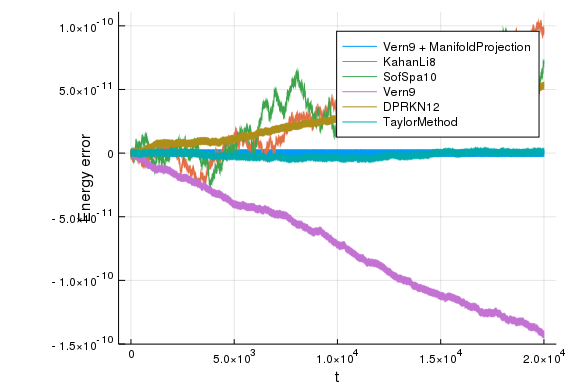As we can see from the above plots, we can achieve a very low energy error for long time simulation by manifold projection and with very high order Taylor methods. In comparison with the Hénon-Heiles system we see that as the Hamiltonian got more complex, the energy error for the other integration methods increased significantly.

We will now compare the in place with the out of place versions. In the plots bellow we will use a dashed line for the out of place versions.

function in_vs_out(;all=false, tmax=1e2)
println("In place versions:")
plt = compare(InPlace, all, tmax=tmax)
println("\nOut of place versions:")
plt = compare(OutOfPlace, false, plt; tmax=tmax)
end

in_vs_out (generic function with 1 method)


First, here is a summary of all the available methods for tmax = 1e3:

in_vs_out(all=true, tmax=1e3)

In place versions:
0.969917 seconds (3.30 M allocations: 285.846 MiB, 36.63% gc time)
0.254735 seconds (1.50 M allocations: 87.745 MiB, 57.35% gc time)
0.370626 seconds (1.50 M allocations: 87.746 MiB, 53.86% gc time)
0.167306 seconds (632.22 k allocations: 67.646 MiB, 46.57% gc time)
0.050140 seconds (467.44 k allocations: 20.215 MiB)
2.279321 seconds (15.32 M allocations: 2.237 GiB, 30.70% gc time)
Vern9 + ManifoldProjection max energy error:	1.9895196601282805e-13	in	9724
5	steps.
KahanLi8 max energy error:			1.0530243343964685e-11	in	100002	steps.
SofSpa10 max energy error:			1.5077716852829326e-11	in	100002	steps.
Vern9 max energy error:				1.0615508472255897e-11	in	48624	steps.
DPRKN12 max energy error:			4.874323167314287e-12	in	18930	steps.
TaylorMethod max energy error:			1.0089706847793423e-12	in	5082	steps.

Out of place versions:
3.890134 seconds (8.21 M allocations: 432.094 MiB, 6.39% gc time)
1.828772 seconds (3.71 M allocations: 198.850 MiB, 5.71% gc time)
5.499864 seconds (9.13 M allocations: 474.518 MiB, 5.09% gc time)
2.359569 seconds (4.54 M allocations: 225.389 MiB, 5.21% gc time)
KahanLi8 max energy error:			2.2353674467012752e-11	in	100002	steps.
SofSpa10 max energy error:			4.079936388734495e-11	in	100002	steps.
Vern9 max energy error:				5.9259264162392356e-12	in	48632	steps.
DPRKN12 max energy error:			5.3859139370615594e-12	in	18925	steps.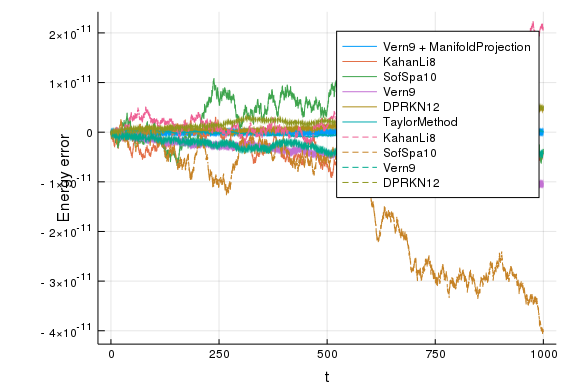Now we will compare the in place and the out of place versions, but only for the integrators that are compatible with StaticArrays

in_vs_out(tmax=1e2)

In place versions:
0.009295 seconds (150.11 k allocations: 8.322 MiB)
0.013982 seconds (150.11 k allocations: 8.323 MiB)
0.007019 seconds (63.33 k allocations: 6.835 MiB)
0.004803 seconds (47.22 k allocations: 2.074 MiB)
KahanLi8 max energy error:			5.5706550483591855e-12	in	10001	steps.
SofSpa10 max energy error:			3.836930773104541e-12	in	10001	steps.
Vern9 max energy error:				1.6058265828178264e-12	in	4864	steps.
DPRKN12 max energy error:			7.105427357601002e-13	in	1907	steps.

Out of place versions:
0.004514 seconds (20.07 k allocations: 1.989 MiB)
0.008056 seconds (20.07 k allocations: 1.990 MiB)
0.005109 seconds (9.83 k allocations: 2.532 MiB)
0.003518 seconds (10.05 k allocations: 798.672 KiB)
KahanLi8 max energy error:			5.229594535194337e-12	in	10001	steps.
SofSpa10 max energy error:			4.007461029686965e-12	in	10001	steps.
Vern9 max energy error:				1.5489831639570184e-12	in	4867	steps.
DPRKN12 max energy error:			9.521272659185342e-13	in	1906	steps.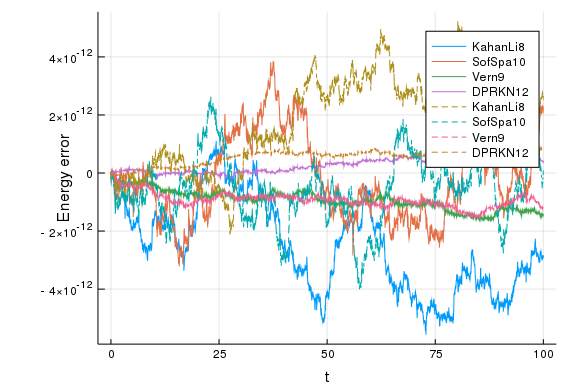in_vs_out(tmax=1e3)

In place versions:
0.219100 seconds (1.50 M allocations: 87.745 MiB, 49.33% gc time)
0.355341 seconds (1.50 M allocations: 87.746 MiB, 51.48% gc time)
0.140694 seconds (632.22 k allocations: 67.646 MiB, 38.72% gc time)
0.050146 seconds (467.44 k allocations: 20.215 MiB)
KahanLi8 max energy error:			1.0530243343964685e-11	in	100002	steps.
SofSpa10 max energy error:			1.5077716852829326e-11	in	100002	steps.
Vern9 max energy error:				1.0615508472255897e-11	in	48624	steps.
DPRKN12 max energy error:			4.874323167314287e-12	in	18930	steps.

Out of place versions:
0.056768 seconds (200.08 k allocations: 25.946 MiB, 10.06% gc time)
0.087501 seconds (200.08 k allocations: 25.947 MiB, 8.54% gc time)
0.056303 seconds (97.37 k allocations: 23.997 MiB, 11.48% gc time)
0.033284 seconds (98.63 k allocations: 7.092 MiB)
KahanLi8 max energy error:			2.2353674467012752e-11	in	100002	steps.
SofSpa10 max energy error:			4.079936388734495e-11	in	100002	steps.
Vern9 max energy error:				5.9259264162392356e-12	in	48632	steps.
DPRKN12 max energy error:			5.3859139370615594e-12	in	18925	steps.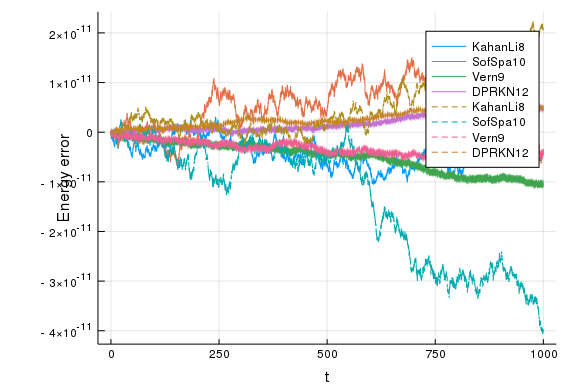in_vs_out(tmax=1e4)

In place versions:
2.210512 seconds (15.00 M allocations: 831.610 MiB, 46.35% gc time)
4.704687 seconds (15.00 M allocations: 831.611 MiB, 63.30% gc time)
5.640583 seconds (6.32 M allocations: 676.280 MiB, 83.79% gc time)
4.261226 seconds (4.67 M allocations: 199.615 MiB, 87.98% gc time)
KahanLi8 max energy error:			4.3968384488835e-11	in	1000001	steps.
SofSpa10 max energy error:			6.492939519375795e-11	in	1000001	steps.
Vern9 max energy error:				7.220535280794138e-11	in	486181	steps.
DPRKN12 max energy error:			2.701483481359901e-11	in	189210	steps.

Out of place versions:
0.719979 seconds (2.00 M allocations: 198.370 MiB, 30.92% gc time)
1.306247 seconds (2.00 M allocations: 198.371 MiB, 40.31% gc time)
0.878160 seconds (972.55 k allocations: 235.100 MiB, 43.56% gc time)
0.417349 seconds (984.51 k allocations: 61.433 MiB, 20.86% gc time)
KahanLi8 max energy error:			3.595346242946107e-11	in	1000001	steps.
SofSpa10 max energy error:			8.72120153871947e-11	in	1000001	steps.
Vern9 max energy error:				6.254197160160402e-11	in	486217	steps.
DPRKN12 max energy error:			2.41158204516978e-11	in	189176	steps.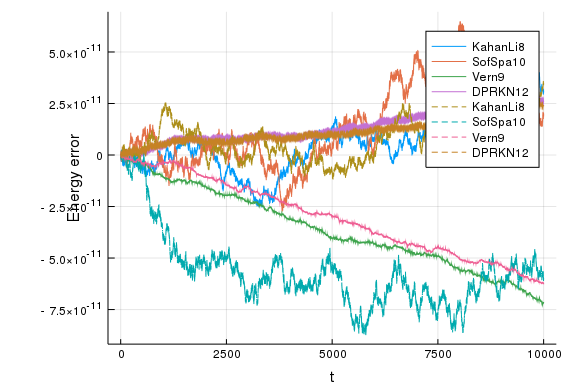in_vs_out(tmax=2e4)

In place versions:
4.239649 seconds (30.00 M allocations: 1.669 GiB, 43.69% gc time)
10.526999 seconds (30.00 M allocations: 1.669 GiB, 66.65% gc time)
13.394114 seconds (12.64 M allocations: 1.316 GiB, 86.29% gc time)
7.997147 seconds (9.34 M allocations: 394.505 MiB, 87.24% gc time)
KahanLi8 max energy error:			1.0363976343796821e-10	in	2000002	steps.
SofSpa10 max energy error:			9.750067420100095e-11	in	2000002	steps.
Vern9 max energy error:				1.4270540305005852e-10	in	972360	steps.
DPRKN12 max energy error:			5.31912291990011e-11	in	378394	steps.

Out of place versions:
1.416756 seconds (4.00 M allocations: 488.287 MiB, 29.14% gc time)
2.564736 seconds (4.00 M allocations: 488.288 MiB, 38.30% gc time)
1.858632 seconds (1.94 M allocations: 465.496 MiB, 46.71% gc time)
1.225899 seconds (1.97 M allocations: 118.165 MiB, 46.04% gc time)
KahanLi8 max energy error:			4.359890226623975e-11	in	2000002	steps.
SofSpa10 max energy error:			8.72120153871947e-11	in	2000002	steps.
Vern9 max energy error:				1.2143175354140112e-10	in	972435	steps.
DPRKN12 max energy error:			6.365041826938977e-11	in	378345	steps.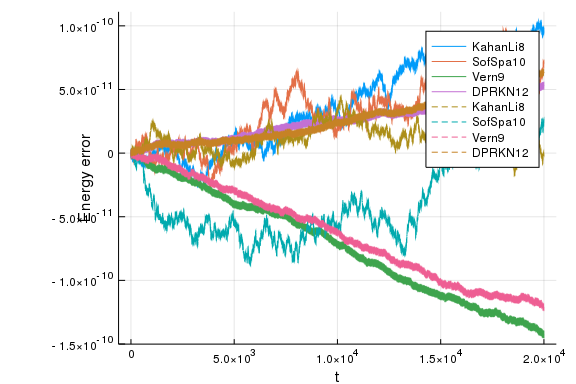As we see from the above comparisons, the StaticArray versions are significantly faster and use less memory. The speedup provided for the out of place version is more proeminent at larger values for tmax. We can see again that if the simulation time is increased, the energy error of the symplectic methods is less noticeable compared to the rest of the methods. In comparison with the Henon-Heiles case, we see that the symplectic methods are more competitive with DPRKN12.

using DiffEqBenchmarks
DiffEqBenchmarks.bench_footer(WEAVE_ARGS[:folder],WEAVE_ARGS[:file])


## Appendix

These benchmarks are a part of the DiffEqBenchmarks.jl repository, found at: https://github.com/JuliaDiffEq/DiffEqBenchmarks.jl

To locally run this tutorial, do the following commands:

using DiffEqBenchmarks
DiffEqBenchmarks.weave_file("DynamicalODE","Quadrupole_boson_Hamiltonian_energy_conservation_benchmark.jmd")

Computer Information:

Julia Version 1.1.0
Commit 80516ca202 (2019-01-21 21:24 UTC)
Platform Info:
OS: Linux (x86_64-pc-linux-gnu)
CPU: Intel(R) Xeon(R) CPU E5-2680 v4 @ 2.40GHz
WORD_SIZE: 64
LIBM: libopenlibm
LLVM: libLLVM-6.0.1 (ORCJIT, haswell)


Package Information:

Status: /home/crackauckas/.julia/environments/v1.1/Project.toml
[c52e3926-4ff0-5f6e-af25-54175e0327b1] Atom 0.8.5
[bcd4f6db-9728-5f36-b5f7-82caef46ccdb] DelayDiffEq 5.2.0
[bb2cbb15-79fc-5d1e-9bf1-8ae49c7c1650] DiffEqBenchmarks 0.1.0
[459566f4-90b8-5000-8ac3-15dfb0a30def] DiffEqCallbacks 2.5.2
[f3b72e0c-5b89-59e1-b016-84e28bfd966d] DiffEqDevTools 2.7.2
[78ddff82-25fc-5f2b-89aa-309469cbf16f] DiffEqMonteCarlo 0.14.0
[77a26b50-5914-5dd7-bc55-306e6241c503] DiffEqNoiseProcess 3.1.0
[055956cb-9e8b-5191-98cc-73ae4a59e68a] DiffEqPhysics 3.1.0
[a077e3f3-b75c-5d7f-a0c6-6bc4c8ec64a9] DiffEqProblemLibrary 4.1.0
[0c46a032-eb83-5123-abaf-570d42b7fbaa] DifferentialEquations 6.3.0
[b305315f-e792-5b7a-8f41-49f472929428] Elliptic 0.5.0
[e5e0dc1b-0480-54bc-9374-aad01c23163d] Juno 0.7.0
[7f56f5a3-f504-529b-bc02-0b1fe5e64312] LSODA 0.4.0
[c030b06c-0b6d-57c2-b091-7029874bd033] ODE 2.4.0
[54ca160b-1b9f-5127-a996-1867f4bc2a2c] ODEInterface 0.4.5
[09606e27-ecf5-54fc-bb29-004bd9f985bf] ODEInterfaceDiffEq 3.1.0
[1dea7af3-3e70-54e6-95c3-0bf5283fa5ed] OrdinaryDiffEq 5.6.0
[2dcacdae-9679-587a-88bb-8b444fb7085b] ParallelDataTransfer 0.5.0
[65888b18-ceab-5e60-b2b9-181511a3b968] ParameterizedFunctions 4.1.1
[91a5bcdd-55d7-5caf-9e0b-520d859cae80] Plots 0.24.0
[d330b81b-6aea-500a-939a-2ce795aea3ee] PyPlot 2.8.1
[90137ffa-7385-5640-81b9-e52037218182] StaticArrays 0.10.3
[789caeaf-c7a9-5a7d-9973-96adeb23e2a0] StochasticDiffEq 6.1.1
[c3572dad-4567-51f8-b174-8c6c989267f4] Sundials 3.4.0
[92b13dbe-c966-51a2-8445-caca9f8a7d42] TaylorIntegration 0.4.1
[44d3d7a6-8a23-5bf8-98c5-b353f8df5ec9] Weave 0.9.0